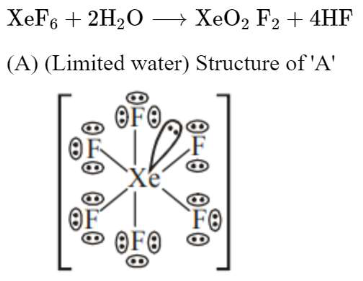# A xenon compound 'A' upon partial hydrolysis givesQuestion:

A xenon compound 'A' upon partial hydrolysis gives $\mathrm{XeO}_{2} \mathrm{~F}_{2}$. The number of lone pair of electrons present in compound $\mathrm{A}$ is_______________ .

(Round off to the Nearest integer)

Solution:

(19)Total l.p. on $(\mathrm{A})=19$